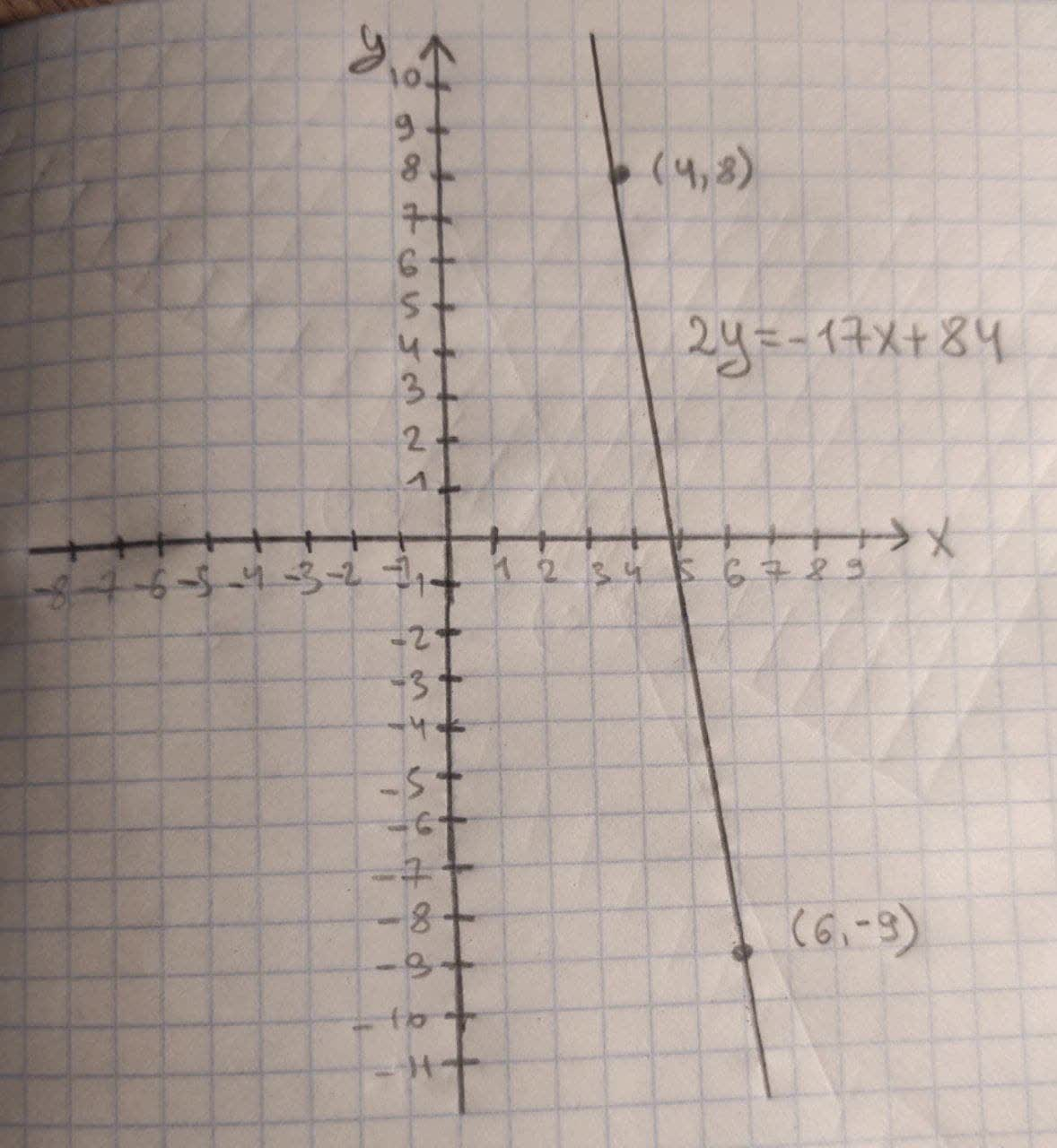What would be the linear equation for this function, & the graph?(X_1,Y_1)=(4,8), (X_2,Y_2)=(6,-9)Wribreeminsl 2020-10-23 Answered

What would be the linear equation for this function, and the graph?
$$\displaystyle{\left({X}_{{1}},{Y}_{{1}}\right)}={\left({4},{8}\right)},{\left({X}_{{2}},{Y}_{{2}}\right)}={\left({6},-{9}\right)}$$

• Questions are typically answered in as fast as 30 minutes

Solve your problem for the price of one coffee

• Math expert for every subject
• Pay only if we can solve itSadie Eaton
Formula used:
Point-slope form of line:
$$\displaystyle{\left({y}_{{2}}-{y}_{{1}}\right)}={m}{\left({x}_{{2}}-{x}_{{1}}\right)}$$
So, by the Point-Slope form of line the equation of line is given below:
$$\displaystyle{\left(-{9}-{8}\right)}={m}{\left({6}-{4}\right)}$$
$$\displaystyle-{17}={m}\times{2}$$
$$\displaystyle{2}{m}=-{17}$$
$$\displaystyle{m}=-\frac{{17}}{{2}}$$
Now $$\displaystyle{m}=-\frac{{17}}{{2}}$$ and take any points to find the linear equation.
$$\displaystyle{\left({x}_{{1}},{y}_{{1}}\right)}={\left({4},{8}\right)}{\quad\text{and}\quad}{m}=-\frac{{17}}{{2}}$$
$$\displaystyle{\left({y}-{y}_{{1}}\right)}={m}{\left({x}-{x}_{{1}}\right)}$$
$$\displaystyle{\left({y}-{8}\right)}=-\frac{{17}}{{2}}{\left({x}-{4}\right)}$$
$$\displaystyle{\left({y}-{8}\right)}=\frac{{-{17}{x}}}{{2}}+{34}$$
$$\displaystyle{y}=\frac{{-{17}{x}}}{{2}}+{34}+{8}$$
$$\displaystyle{y}=\frac{{-{17}{x}}}{{2}}+{42}$$
$$\displaystyle{y}=\frac{{-{17}{x}+{84}}}{{2}}$$
$$\displaystyle{2}{y}=-{17}{x}_{{84}}$$
So, the graph is given below: